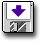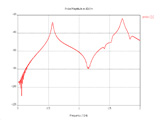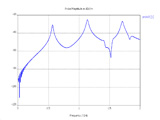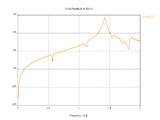Modeling Powerbus with CST

 Geometry and setup Double-sided PCB: Size: 125 mm *100 mm *1 mm Top and bottom metal: PEC Dielectric: FR4, εr = 4.5, loss tangent = 0.015 @ 1 GHz (Specification: Const. fit tan delta) Model parameters: Frequency: 5 MHz - 2 GHz Steady state accuracy limit: -50 dB Mesh definition: Mesh type: Hexahedral Mesh density control: Lines per wavelength = 40, Others = Default Special mesh properties: Refine at PEC/lossy metal edges by factor = 6, Others = Default Automesh Excitation: Discrete port (1 V, 50 ohms)cst_powerbus.zip Simulation result Simulation time: 38 mins, 43 secs Number of mesh cells: 248864 Excitation duration: 3.563457e+000 ns Calculation time for excitation: 152 secs Number of calculated pulse widths: 19.9997 Simulated number of time steps: 62402 Maximum number of time steps: 62402 Decisions the user must make that affect the accuracy of the result Mesh density control: the minimum Lines per wavelength is 40. Special mesh properties: 6 or greater value is needed for 'Refine at PEC/lossy metal edges by factor' to obtain higher accuracy. Comments What source do we use in this example? In this example, we build two models to get all the results. One model uses 'S-Parameter' port type with impedance of 50 ohms to get the input impedance. The other model uses 'Voltage' port type with voltage of 1.0 volts connected with a 50 ohms impedance in series to get the electric field. Can CST model dielectric materials with constant loss tangent?CST cannot model materials with constant loss tangent. In this model, we model the dielectrics having a loss tangent of 0.015 at 1 GHz and select the specification of 'Const. fit tan delta'.More information...
 Screen shotsFig. 4. Electric field at 3 m, theta=0o, phi=0oFig. 5. Electric field at 3 m, theta=90o, phi=0oFig. 6. Electric field at 3 m, theta=90o, phi=90o